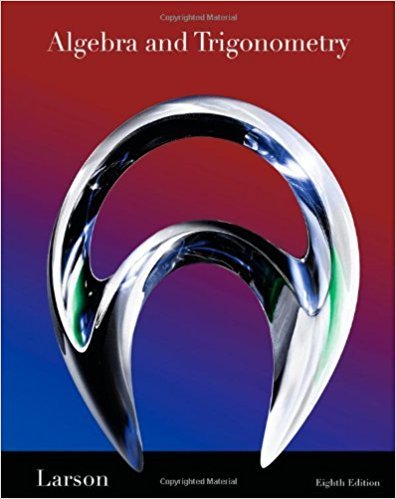×
×

# Solutions for Chapter P.1: Review of Real Numbers and Their Properties## Full solutions for Algebra and Trigonometry | 8th Edition

ISBN: 9781439048474Solutions for Chapter P.1: Review of Real Numbers and Their Properties

Solutions for Chapter P.1
4 5 0 381 Reviews
12
3
##### ISBN: 9781439048474

Algebra and Trigonometry was written by and is associated to the ISBN: 9781439048474. This textbook survival guide was created for the textbook: Algebra and Trigonometry, edition: 8. Chapter P.1: Review of Real Numbers and Their Properties includes 134 full step-by-step solutions. This expansive textbook survival guide covers the following chapters and their solutions. Since 134 problems in chapter P.1: Review of Real Numbers and Their Properties have been answered, more than 51797 students have viewed full step-by-step solutions from this chapter.

Key Math Terms and definitions covered in this textbook
• Big formula for n by n determinants.

Det(A) is a sum of n! terms. For each term: Multiply one entry from each row and column of A: rows in order 1, ... , nand column order given by a permutation P. Each of the n! P 's has a + or - sign.

A sequence of steps (end of Chapter 9) to solve positive definite Ax = b by minimizing !x T Ax - x Tb over growing Krylov subspaces.

• Cramer's Rule for Ax = b.

B j has b replacing column j of A; x j = det B j I det A

• Cyclic shift

S. Permutation with S21 = 1, S32 = 1, ... , finally SIn = 1. Its eigenvalues are the nth roots e2lrik/n of 1; eigenvectors are columns of the Fourier matrix F.

• Fast Fourier Transform (FFT).

A factorization of the Fourier matrix Fn into e = log2 n matrices Si times a permutation. Each Si needs only nl2 multiplications, so Fnx and Fn-1c can be computed with ne/2 multiplications. Revolutionary.

• Fibonacci numbers

0,1,1,2,3,5, ... satisfy Fn = Fn-l + Fn- 2 = (A7 -A~)I()q -A2). Growth rate Al = (1 + .J5) 12 is the largest eigenvalue of the Fibonacci matrix [ } A].

• Free columns of A.

Columns without pivots; these are combinations of earlier columns.

• Free variable Xi.

Column i has no pivot in elimination. We can give the n - r free variables any values, then Ax = b determines the r pivot variables (if solvable!).

• Incidence matrix of a directed graph.

The m by n edge-node incidence matrix has a row for each edge (node i to node j), with entries -1 and 1 in columns i and j .

• Kronecker product (tensor product) A ® B.

Blocks aij B, eigenvalues Ap(A)Aq(B).

• Least squares solution X.

The vector x that minimizes the error lie 112 solves AT Ax = ATb. Then e = b - Ax is orthogonal to all columns of A.

• Nilpotent matrix N.

Some power of N is the zero matrix, N k = o. The only eigenvalue is A = 0 (repeated n times). Examples: triangular matrices with zero diagonal.

• Nullspace matrix N.

The columns of N are the n - r special solutions to As = O.

• Permutation matrix P.

There are n! orders of 1, ... , n. The n! P 's have the rows of I in those orders. P A puts the rows of A in the same order. P is even or odd (det P = 1 or -1) based on the number of row exchanges to reach I.

• Pseudoinverse A+ (Moore-Penrose inverse).

The n by m matrix that "inverts" A from column space back to row space, with N(A+) = N(AT). A+ A and AA+ are the projection matrices onto the row space and column space. Rank(A +) = rank(A).

• Singular matrix A.

A square matrix that has no inverse: det(A) = o.

• Singular Value Decomposition

(SVD) A = U:E VT = (orthogonal) ( diag)( orthogonal) First r columns of U and V are orthonormal bases of C (A) and C (AT), AVi = O'iUi with singular value O'i > O. Last columns are orthonormal bases of nullspaces.

• Sum V + W of subs paces.

Space of all (v in V) + (w in W). Direct sum: V n W = to}.

• Tridiagonal matrix T: tij = 0 if Ii - j I > 1.

T- 1 has rank 1 above and below diagonal.

• Vector space V.

Set of vectors such that all combinations cv + d w remain within V. Eight required rules are given in Section 3.1 for scalars c, d and vectors v, w.

×